Refer to our Texas Go Math Grade 2 Answer Key Pdf to score good marks in the exams. Test yourself by practicing the problems from Texas Go Math Grade 2 Lesson 13.4 Answer Key Addition Situations.

Unlock the Problem

Kendra had 35 crayons. Her dad gave her some more crayons. Then she had 49 crayons. How many crayons did Kendra’s dad give her?

The number of crayons did Kendra’s dad give her is 14 crayons.

Explanation:
Given that Kendra had 35 crayons and her dad gave her some more crayons, then she had 49 crayons. So the number of crayons did Kendra’s dad give her is 49 – 35 = 14 crayons.

What information am I given?
he gave her some more crayons, the had ______ crayons.

he gave her some more crayons, the had 49 crayons.

Plan

What ¡s my plan or strategy?
I can ___________ to solve the problem.

I can subtract to solve the problem.

Solve

Show how you solve the problem.___________ crayons
35 + 14 = 49 crayons.

Explanation:
As Kendra’s had 35 crayons and her dad gave her 14 crayons, so the total number of crayons does Kendra had is 35 + 14 which 49 crayons.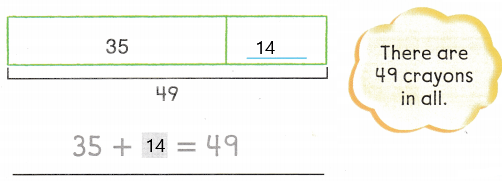HOME CONNECTION • Your child used a model and a number sentence to represent the problem. These help show what the missing amount is ¡n order to solve the problem.

Try Another Problem

Label the model. Write a number sentence with a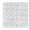for the unknown number. Solve.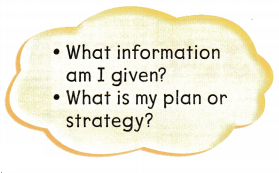Mr. Kane has 104- red pens. He bought 19 blue pens. How many pens does he have now?___________ pens
The number of pens does he have now is 123 pens.

Explanation:
Given Mr.Kane has 104- red pens and he bought 19 blue pens. So the number of pens does he have now is 104 + 19 which is 123 pens.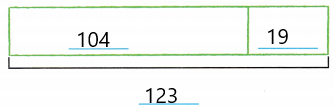Question 2.
Jim has some pencils. Hannah has 13 pencils. They have 31 pencils altogether. How many pencils does Jim have?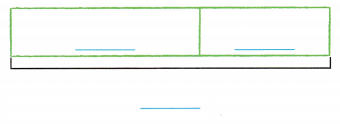___________ pencils
Jim has 18 pencils.

Explanation:
Given that Jim has some pencils and Hannah has 13 pencils. They have 31 pencils altogether, so the number of pencils does Jim has is 31 – 13 = 18 pencils.Math Talk
Mathematical Processes

Explain how you know if amount is a part or the whole in a problem.

Share and Show

Label the model. Write a number sentence with afor the unknown number. Solve.

Question 3.
Aimee and Matthew catch 17 crickets in all. Aimee catches 9 crickets. How many crickets does Matthew catch?__________ crickets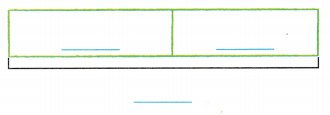The number of crickets does Matthew caught is 26 crickets.

Explanation:
Given that Aimee and Matthew catch 17 crickets in all and Aimee catches 9 crickets. So the number of crickets does Matthew caught is 17 + 9 which is 26 crickets.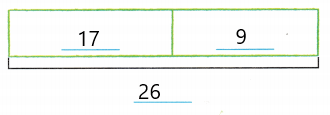Problem Solving

Solve. Write or draw to show your work.

Question 4.
H.O.T. Multi-Step There are three groups of owls. There are 17 owls in each of the first two groups. There are 53 owls in all. How many owls are in the third group?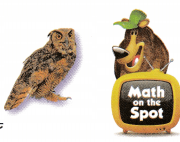__________ owls
The number of owls in the third group will be 19 owls.

Explanation:
Given that there are 17 owls in each of the first two groups, so the owls in the first two groups are 17 + 17 which is 34. And there are 53 owls in all. So the number of owls in the third group will be 53 – 34 which is 19 owls.

H.O.T. Percy sees a grasshopper jumping in the grass. He counts 16 jumps. 9 jumps, and then 15 more jumps. How many jumps does he count?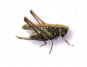_________ jumps
The number of jumps does Percy counts is 40 jumps.

Explanation:
Given that Percy sees a grasshopper jumping in the grass and he counts 16 jumps, 9 jumps, and then 15 more jumps. So the number of jumps does he count is 16 + 9 + 15 which is 40 jumps.

Question 6.
Analyze The first book has 79 pages. The second book has 67 pages. How many pages are in these books?
(A) 146
(B) 136
(C) 149
A.

Explanation:
Given that the first book has 79 pages and the second book has 67 pages. So the number of pages are in these books is 79 + 67 which is 146.

Question 7.
Mr. Green saw 60 books on a shelf and some books on a table. He saw 140 books in all. How many books did Mr. Green see on the table?
Write a number sentence with afor the unknown number. Then solve.
______________ books
The number of books did Mr. Green saw is 80 books.

Explanation:
Given that Mr. Green saw 60 books on a shelf and some books on a table and He saw 140 books in all. So the number of books did Mr. Green saw on the table is 140 – 60 which is 80 books.

Question 8.
To solve for the missing number, describe a strategy that you could use.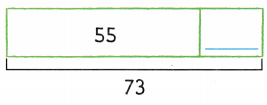The missing number is 18.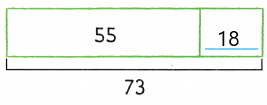Texas Test Prep There are 23 books in a box. There are 29 books on a shelf. How many books are there?
(A) 52
(B) 56
(C) 42
52 books.

Explanation:
Given that there are 23 books in a box and there are 29 books on a shelf. So the number of books are 23 + 29 which is 52 books.

TAKE HOME ACTIVITY • Ask your child to explain how to solve one of the problems in this lesson.

### Texas Go Math Grade 2 Lesson 13.4 Homework and Practice Answer Key

Label the model. Write a number sentence with afor the unknown number. Solve.

Question 1.
June and Mike catch 15 fish in all. June catches 8 fish. How many fish does Mike catch?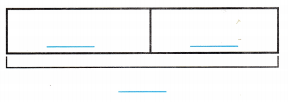_________ fish
The number of fish did Mike caught is 7 fish.

Explanation:
Given that June and Mike catch 15 fish in all and June catches 8 fish. So the number of fishes did Mike caught is 15 – 8 which is 7 fish.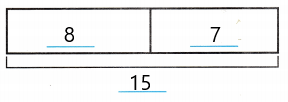Problem Solving

Solve. Write or draw to show your work.

Question 2.
Pam sees 12 frogs jumping near a pond. She also sees, 7 turtles, and then 17 rabbits. How many animals does she see?
__________ animals
The animals did she saw is 36 animals.

Explanation:
Given that Pam sees 12 frogs jumping near a pond and she also sees, 7 turtles, and then 17 rabbits. So the number of animals she saw is 12 + 7 + 17 which is 36 animals.

Multi-Step There are a total of 41 chickens on the farm. There are 13 chickens in each of 2 cages in the barn. The rest of the chickens are outside. How many chickens are outside?
___________ chickens
The number of chickens that are outside is 15 chickens.

Explanation:
Given that there are a total of 41 chickens on the farm and there are 13 chickens in each of 2 cages in the barn which is 13×2= 26 chickens. So the number of chickens are outside is 41 – 26 which is 15 chickens.

Lesson Check

Question 4.
There are 25 toy cars in a box. There are 37 toy cars on a shelf. How many toy cars are there in all?
(A) 52
(B) 61
(C) 62
C.

Explanation:
Given that there are 25 toy cars in a box and there are 37 toy cars on a shelf. So the total number of cars are there in all is 25 + 37 which is 62 toy cars.

Question 5.
Dennis finds two boxes in the attic. The first box has 53 hats. The second box has 29 hats. How many hats are in the two boxes?
(A) 86
(B) 72
(C) 82
The number of hats is 82 hats.

Explanation:
Given that Dennis finds two boxes in the attic. The first box has 53 hats and the second box has 29 hats, so the total number of hats in the two boxes is 53 + 29  which is 82 hats.

Go Math 2nd Grade Practice and Homework Lesson 13.4 Answer Key Question 6.
Mr. and Mrs. Gray went apple picking. Mr. Gray picked some green apples. Mrs. Gray picked 70 red apples. They picked 160 apples in all. How many green apples did Mr. Gray pick?
(A) 230
(B) 90
(C) 100
Mr. Gray picked 90 green apples.

Explanation:
Given that Mr. Gray picked some green apples and Mrs. Gray picked 70 red apples. They picked 160 apples in all. So the number of green apples did Mr. Gray picked is 160 – 70 which is 90 green apples.

Question 7.
Marcy bought two packages of paper. One package has 88 sheets of paper. The other has 64 sheets. How many sheets of paper did Marcy buy?
(A) 152
(B) 142
(C) 154